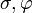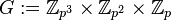# Series-equivalent not implies automorphic in finite abelian group

## Statement

### In terms of subgroups

There can exist a finite abelian group$G$ and subgroups$H$ and$K$ of$G$ such that$H$ and$K$ are series-equivalent subgroups (in other words,$H$ is isomorphic to$K$ and the quotient group$G/H$ is isomorphic to the quotient group$G/K$) but are not automorphic subgroups (i.e., there is no automorphism of$G$ sending$H$ to$K$).

The smallest example for$G$ has order$2^7$, and a similar generic example can be constructed for$p^7$ for any prime number$p$.

### In terms of extensions

There can be a pair of finite abelian groups$A$ and$B$ and two extensions with normal subgroup$A$ and quotient group$B$ such that:

1. The total groups in both extensions are abelian, and are isomorphic groups.
2. The two extensions are not pseudo-congruent extensions, i.e., they cannot be realized as equivalent to each other using automorphisms of$A$ and$B$.

### In terms of cohomology and automorphisms

There can be a pair of finite abelian groups$A$ and$B$ and two elements$\sigma,\varphi$ are elements in the second cohomology group for trivial group action$H^2(B,A)$ such that:

1.$\sigma$ and$\varphi$ are both represented by symmetric 2-cocycles, hence correspond to abelian group extensions.
2. The total groups of the group extensions obtained using the elements$\sigma$ and$\varphi$ are isomorphic as groups.
3.$\sigma$ and$\varphi$ are not in the same orbit of$H^2(B,A)$ under the action of$\operatorname{Aut}(A) \times \operatorname{Aut}(B)$.

### Equivalence of formulations

• Between extensions and subgroups formulations: The formulation in terms of extensions can be interpreted in terms of subgroups as follows: in the first extension$A$ is realized as$H$ and$B$ as$G/H$, and in the second extension,$A$ is realized as$K$ and$B$ as$G/K$. The absence of an automorphism sending$H$ to$K$ is equivalent to the absence of a pseudo-congruence of extensions.
• Between cohomology and extensions formulations: Direct from the interpretation of the second cohomology group in terms of group extensions.

## Related facts

### Weaker facts

Here are some intermediate versions:

Statement Constraint on$G,H,K$ Smallest order of$G$ among known examples Isomorphism class of$G$ Isomorphism class of$H,K$ Isomorphism class of quotient group$G/H, G/K$
series-equivalent abelian-quotient abelian not implies automorphic$H$ and$G/H$ are both abelian 16 nontrivial semidirect product of Z4 and Z4 direct product of Z4 and Z2 cyclic group:Z2
series-equivalent characteristic central subgroups may be distinct$H$ and$K$ are both central subgroups of$G$ 32 SmallGroup(32,28) cyclic group:Z2 direct product of D8 and Z2
series-equivalent abelian-quotient central subgroups not implies automorphic$H$ and$K$ are central and$G/H, G/K$ are abelian 64 semidirect product of Z8 and Z8 of M-type direct product of Z4 and Z2 direct product of Z4 and Z2

### The notion of Hall polynomials

Further information: Hall polynomial

Hall polynomials are polynomials that give a formula for the number of subgroups in an abelian group of prime power order having a particular isomorphism class with a particular isomorphism class for the quotient group.

## Proof

### Example of order$p^6$

We construct an example of an abelian group$G$ of order$p^6$, and subgroups$H$ and$K$ of order$p^3$ such that$H \cong K$ and$G/H \cong G/K$.

We denote by$\mathbb{Z}_n$ the group of integers modulo$n$.$G := \mathbb{Z}_{p^3} \times \mathbb{Z}_{p^2} \times \mathbb{Z}_p$.

We define the subgroups$H$ and$K$ as follows.$\! H = \{ (pa,0,b) \} = p\mathbb{Z}_{p^3} \times 0 \times \mathbb{Z}_p \times \mathbb{Z}_p = \langle (p,0,0), (0,0,1) \rangle$$\! K = \{ (pa,pb,a) \} = langle (p,0,1), (0,p,0) \rangle$

Note that the letter$a$ used in the definition of$K$ should be considered as an integer rather than an integer mod$p$, because its use for the first coordinate requires considering it mod$p^2$.

Then,$H$ and$K$ are both of type$(p^2,p)$, and the quotients$G/H$ and$G/K$ are both of type$(p^2,p)$. Thus,$H \cong K$ and$G/H \cong G/K$.

However, there is no automorphism of$G$ sending$H$ to$K$. For this, note that$H$ contains elements that are$p$ times elements of order$p^3$, but$K$ does not contain any such element.

### Note on dual example

Since subgroup lattice and quotient lattice of finite abelian group are isomorphic, we can invert the above example so as to get both$H$ and$K$ of type$(p^2,p)$ and both$G/H$ and$G/K$ of type$(p^2,p,p)$.